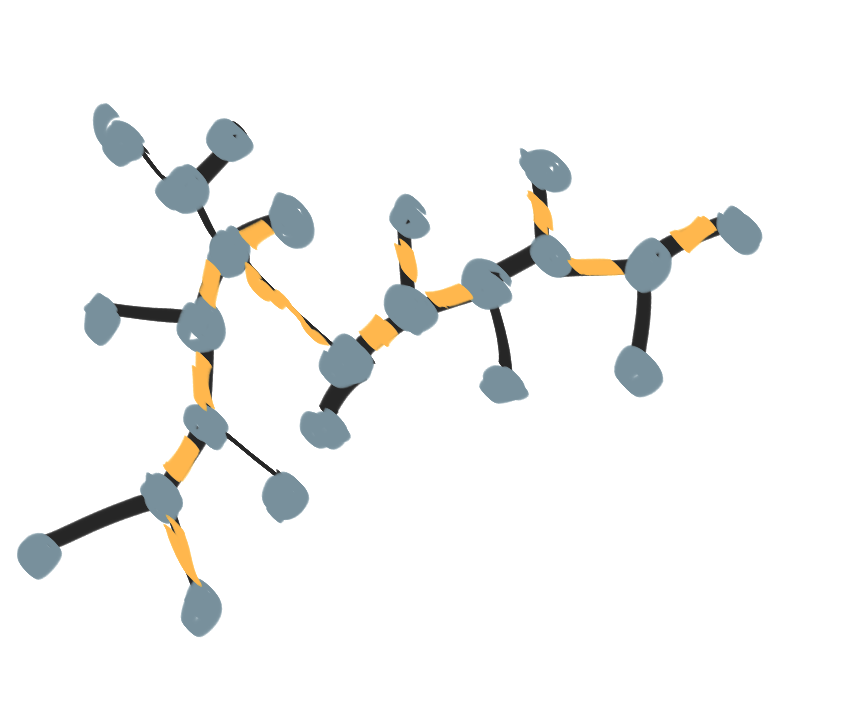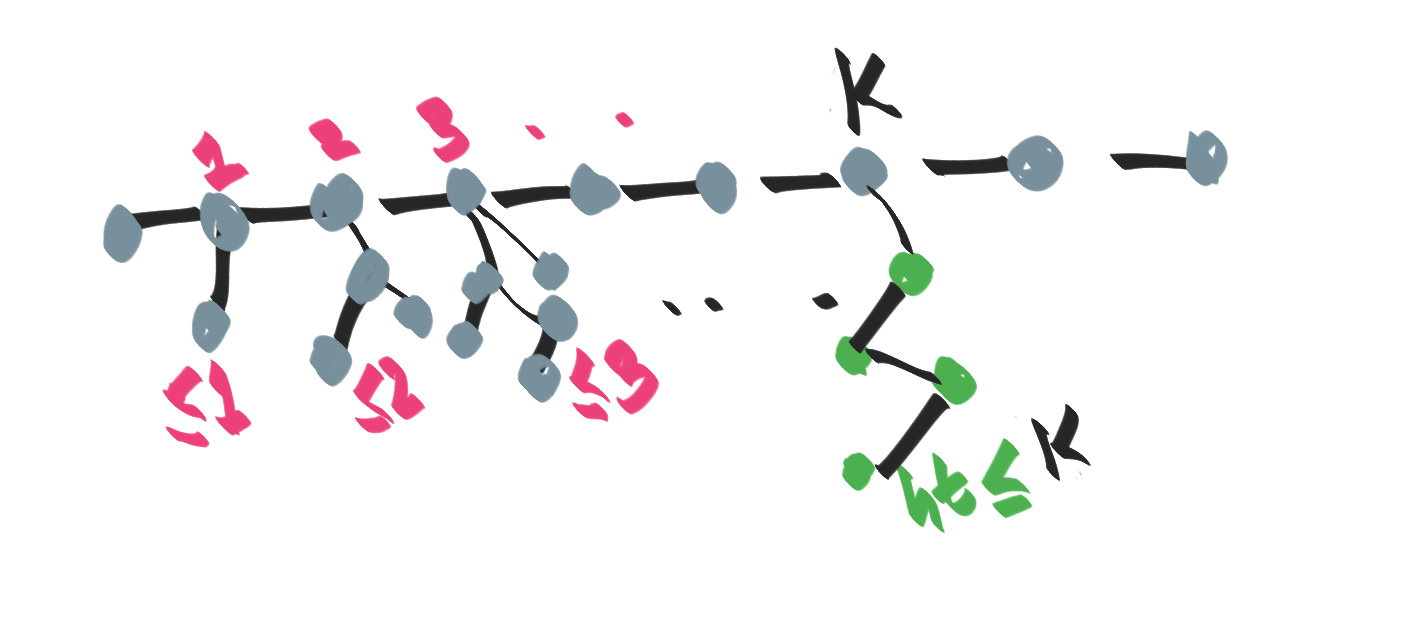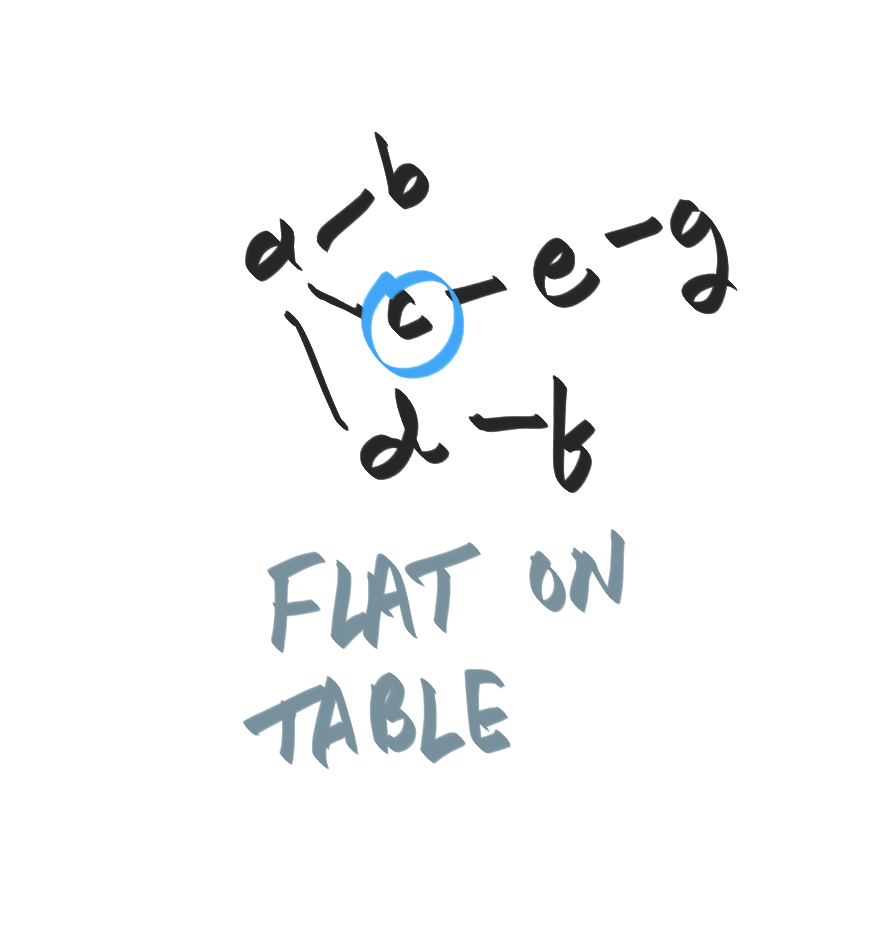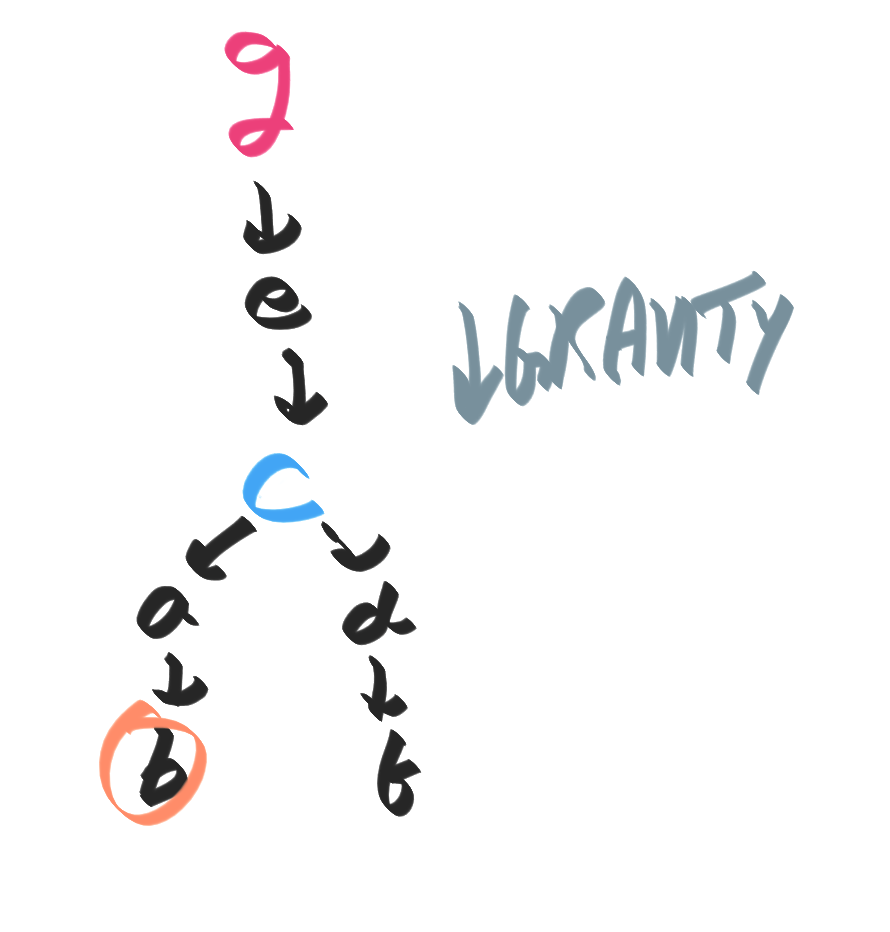## § Diameter of a tree

### § Key property of the diameter

• Let $p$ be a path of maximum diameter, which starts at $p$ and ends at $q$. Consider a tree where the diameter is shown in golden:• We claim that a node at distance $d$ from the left can have a subtree of height at most $d$:• Suppose this were not the case. Then, we can build a longer diameter (in pink) that is longer than the "supposed diameter" (in gold):### § Algorithm to find the diameter:

First perform DFS to find a vertex "on the edge", say $v$. Then perform DFS again starting from this vertex $v$. The farthest vertex from $v$, say $w$ gives us the diameter (the distance from $v$ to $w$)

#### § Proof by intuition/picture:

• first imagine the tree lying flat on the table.• Hold the tree up at node $c$. It's going to fall by gravity and arrange as shown below. This is the same as performing a DFS.• Pick one of the lowest nodes (we pick $g$). Now hold the entire tree from this lowest node, and once again allow gravity to act.• This will give us new lowest nodes such as $b$. This node $b$ is going to be diameter, "because" it's the distance from a lowest node to another lowest node.